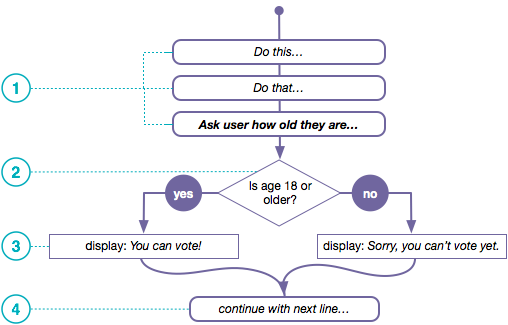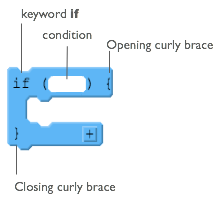# If Statements

## Understanding Program Flow

Programs are said to have a "flow of execution". You start by executing a line of code, then the next, then the next, and so on.A flow chart is a common visual used to represent the various paths of execution that your program might take. Many people use them to help plan programs.

1. This flow chart depicts a program executing one line after another until it gets to a point where it needs to make a decision.
2. In order to determine which path to take you state some condition. It should be a Boolean expression - something that evaluates to true or false. Here we have a simple comparsion of two values: the person's age and the number 18.
3. The program does one thing if the condition is true, and something else if the condition is false.
4. The program can continue a single thread of execution after the condition as well.

## How If-statements workif statements are the lines of code you use to change the flow of a program while it's running. You can write code that determines which lines of code should run next.

At the right is a diagram showing the elements of a basic if statement in JavaScript.

There are two basic parts to an if-statement.

1. A condition to be evaluated (A Boolean expression that evaluates to true or false)
2. Code that should run if the expression was true - enclosed in curly braces

Found a bug in the documentation? Let us know at documentation@code.org